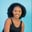Related Tags

golang
strcon
communitycreator

# How to use the strconv.ParseFloat() function in GolangMaria Elijah

### Golang strconv.ParseFloat() function

The ParseFloat() function is a strconv package inbuilt function that converts strings to a floating-point number with the precision defined by the bitSize. The value of bitSize must be 32 or 64. 32 is used for float32, and 64 is used for float64.

Note: The converted result has type float64 when bitSize=32, but it can be converted to float32 without altering its value.

### Syntax

func ParseFloat(s string, bitSize int) (float64, error)


### Parameter

• s: This is the string value to parse into the floating-point number.
• bitSize: This is to specify the precision. The value of bitSize can be 32 or 64.

### Return value

The ParseFloat() function returns the floating-point number converted from the given string.

### Code

The following code shows how to implement the strconv.ParseFloat() function in Golang:

// Golang program
// strconv.ParseFloat() Function

package main

import (
"fmt"
"strconv"
)

func main() {
// delare variable of type string
var str string
// assigning value
str = "40.98"
// ParseFloat() converts variable str to a floating-point number
// with the precision defined by bitSize
// diplays result
fmt.Println(strconv.ParseFloat(str, 32))
fmt.Println(strconv.ParseFloat(str, 64))
fmt.Println()
// reassigning value
str = "-104.23101"
// ParseFloat() converts variable str to a floating-point number
// with the precision defined by bitSize
// diplays result
fmt.Println(strconv.ParseFloat(str, 32))
fmt.Println(strconv.ParseFloat(str, 64))
fmt.Println()

}

Implementation of strconv.ParseFloat() function

### Explanation

• Line 4: We add the main package.

• Lines 6 to 9: We import necessary packages in the Golang project.

• Lines 11 to 21: We define the main() function, variable str of string type, and assign a value to it. We pass the variable in the ParseFloat() function, which converts the str variable to a floating-point number with the precision defined by bitSize.

• Finally, the result is displayed.

RELATED TAGS

golang
strcon
communitycreator

CONTRIBUTORMaria Elijah
RELATED COURSES

View all Courses

Keep Exploring

Learn in-demand tech skills in half the time